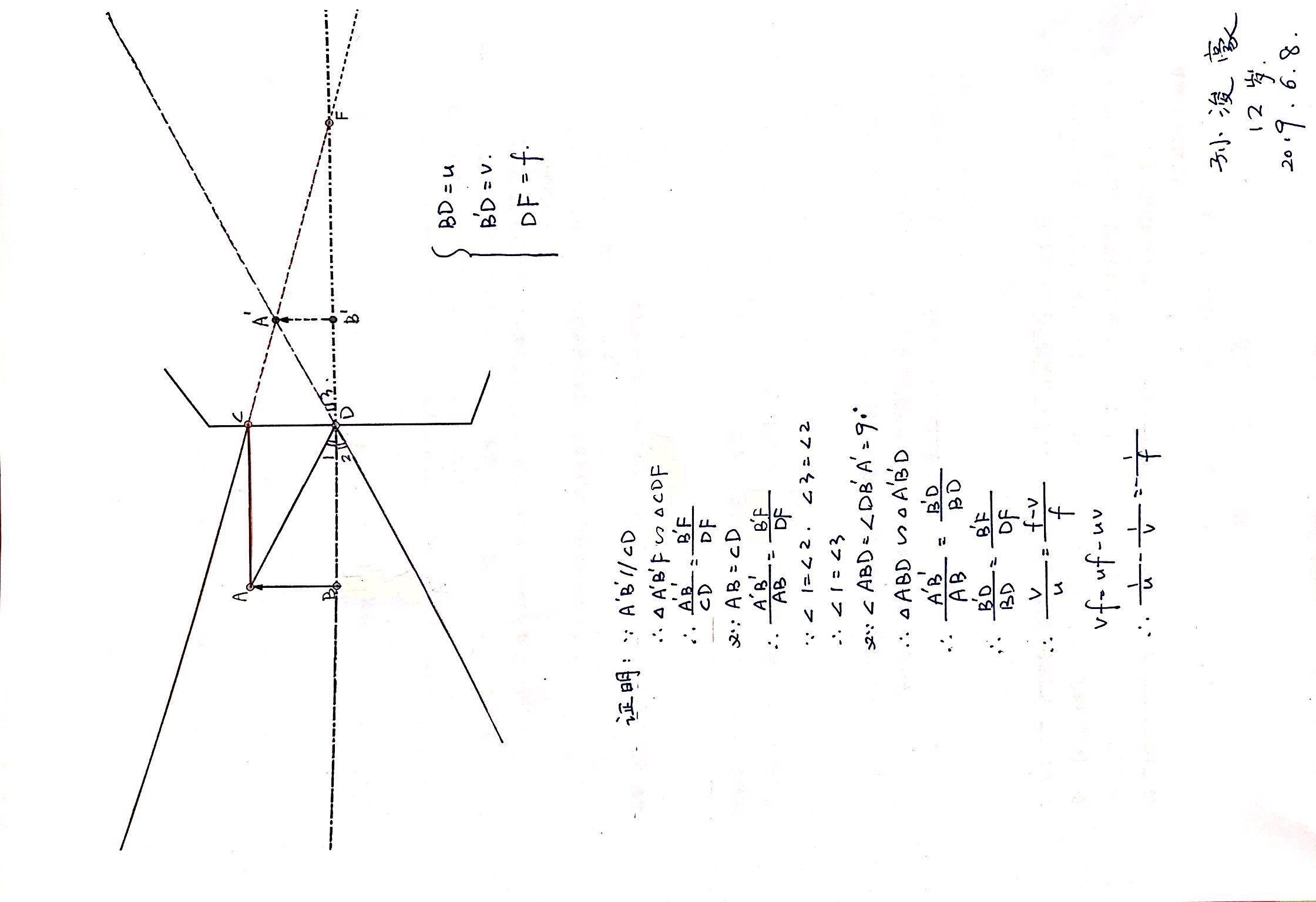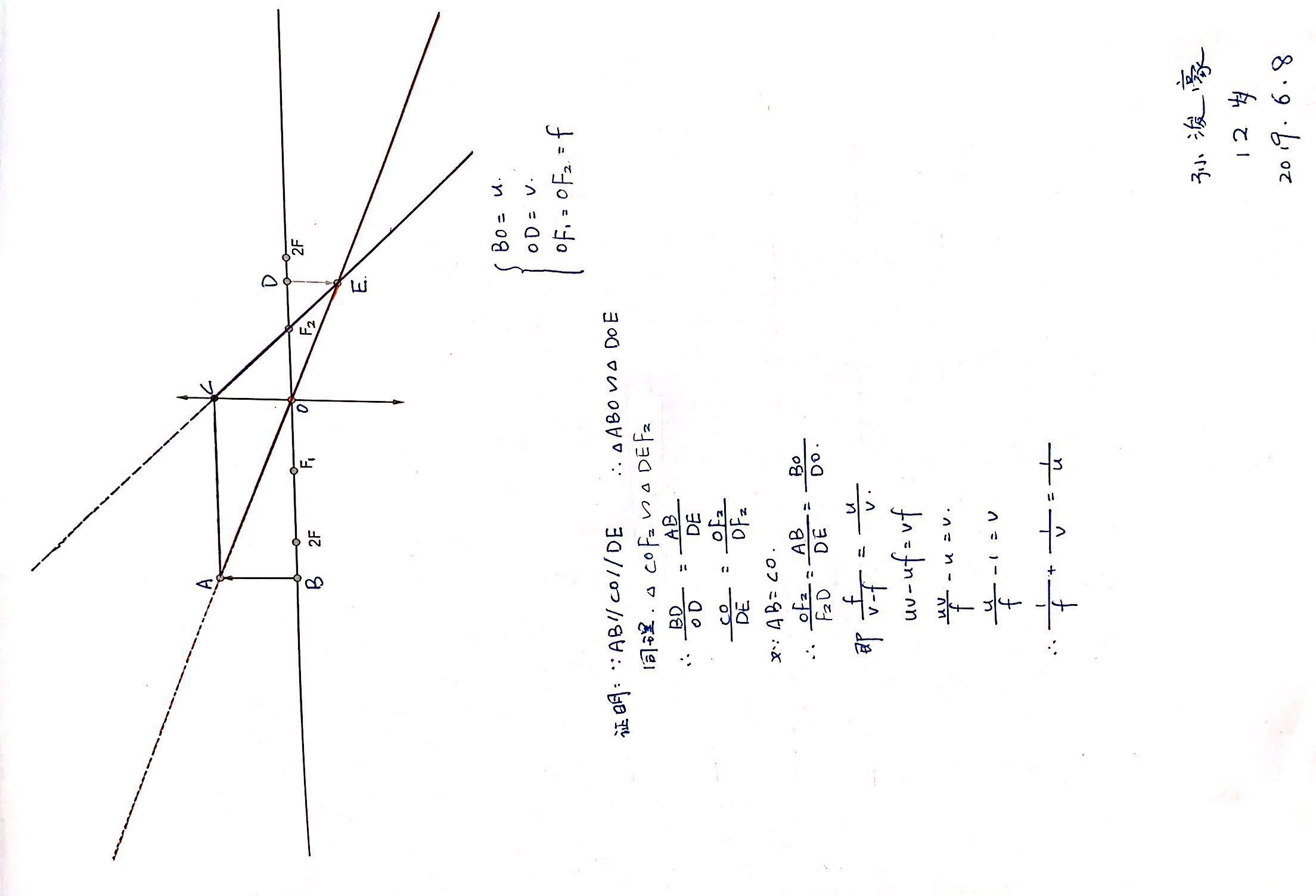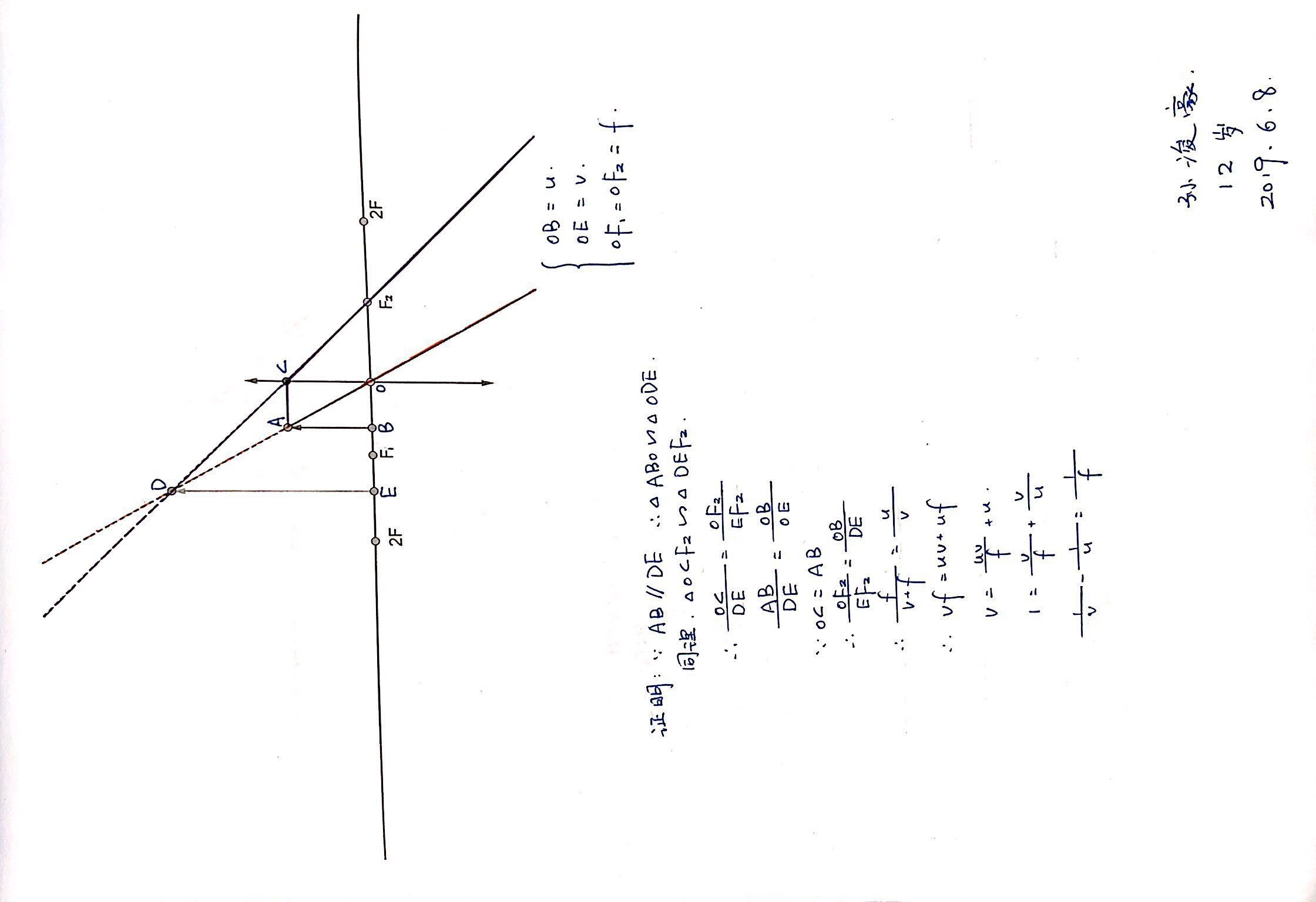05

## 阻力与速度成正比的运动“均匀材料构成的导体圆环，略大于竖直放置的圆柱体。空间存在着垂直于柱体表面的辐射型磁场，圆环所处位置的磁感应强度B，圆环半径为R，质量为m，电阻r，竖直向上的初速度为$v_0$，竖直上抛到回到出发点的过程中总时间，$t_1$$t_2$$q_1$$q_2$$Q_1$$Q_2$、以及$v_\frac{h}{2}$$\frac{v_0}{2}$$\frac{\sqrt{2}}{2}v_0$的大小比较...”$-mgt-\int{kv}dt+\int{kv}dt=-mv_2-mv_1$P.S.有时间应该更新带电粒子在电容里运动的专题整理，特别是那个对称的偏移问题...

10

## 光迹update: